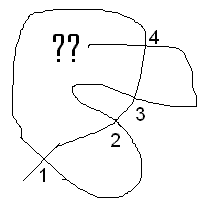#### You may also like### Euler's Officers

How many different ways can you arrange the officers in a square?### FEMTO

A new card game for two players.Follow-up to the February Game Rules of FEMTO.

# Doodles

##### Age 14 to 16 Challenge Level:
James cracked this toughnut for us:

To prove that each vertex number in the list twice, I noticed to get back to where we started pointing in the same direction we started we must go fully around the curve. So we will cover the entire curve, and since the curve goes through each vertex twice, the number of each vertex will appear twice.

For the next part, I tried to doodle with an odd number of vertex number between the pair:As expected, I ran into some problems. If we consider the number between the pair of 1's. Going around anti-clockwise 2 crosses, then 3, then 4, and so on. If there are an odd number of crosses then the end of the line will end up inside the loop. But it can't cross this line again, and so we will always have one end of the line stuck inside the loop, and one outside, and so the curve couldn't be closed.

So between every pair we must have an even number of vertex numbers (since any pair will form a loop similarly to above).